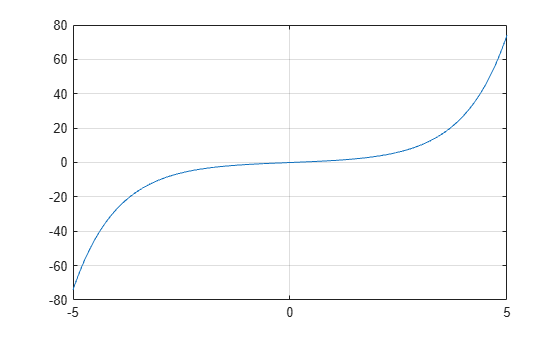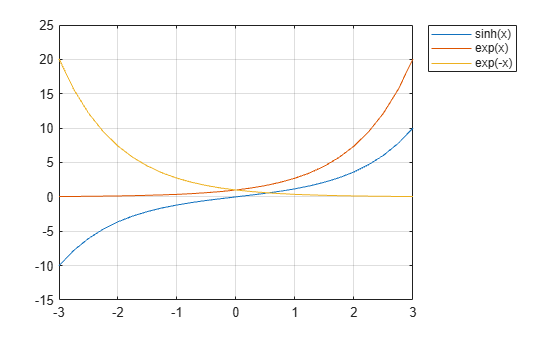# sinh

Hyperbolic sine

## Syntax

``Y = sinh(X)``

## Description

example

````Y = sinh(X)` returns the hyperbolic sine of the elements of `X`. The `sinh` function operates element-wise on arrays. The function accepts both real and complex inputs. All angles are in radians.```

## Examples

collapse all

Create a vector and calculate the hyperbolic sine of each value.

```X = [0 pi 2*pi 3*pi]; Y = sinh(X)```
```Y = 1×4 103 × 0 0.0115 0.2677 6.1958 ```

Plot the hyperbolic sine over the domain $-5\le x\le 5$.

```x = -5:0.01:5; y = sinh(x); plot(x,y) grid on```The hyperbolic sine satisfies the identity $\mathrm{sinh}\left(\mathit{x}\right)=\frac{{\mathit{e}}^{\mathit{x}}-{\mathit{e}}^{-\mathit{x}}}{2}$. In other words, $\mathrm{sinh}\left(\mathit{x}\right)$ is half the difference of the functions ${\mathit{e}}^{\mathit{x}}$ and ${\mathit{e}}^{-\mathit{x}}$. Verify this by plotting the functions.

Create a vector of values between -3 and 3 with a step of 0.25. Calculate and plot the values of `sinh(x)`, `exp(x)`, and `exp(-x)`. As expected, the `sinh` curve is positive where `exp(x)` is large, and negative where `exp(-x)` is large.

```x = -3:0.25:3; y1 = sinh(x); y2 = exp(x); y3 = exp(-x); plot(x,y1,x,y2,x,y3) grid on legend('sinh(x)','exp(x)','exp(-x)','Location','bestoutside')```## Input Arguments

collapse all

Input angles in radians, specified as a scalar, vector, matrix, or multidimensional array.

Data Types: `single` | `double`
Complex Number Support: Yes

collapse all

### Hyperbolic Sine

The hyperbolic sine of an angle x can be expressed in terms of exponential functions as

`$\mathrm{sinh}\left(x\right)=\frac{{e}^{x}-{e}^{-x}}{2}.$`

In terms of the traditional sine function with a complex argument, the identity is

`$\mathrm{sinh}\left(x\right)=-i\mathrm{sin}\left(ix\right)\text{\hspace{0.17em}}.$`

## Version History

Introduced before R2006a